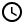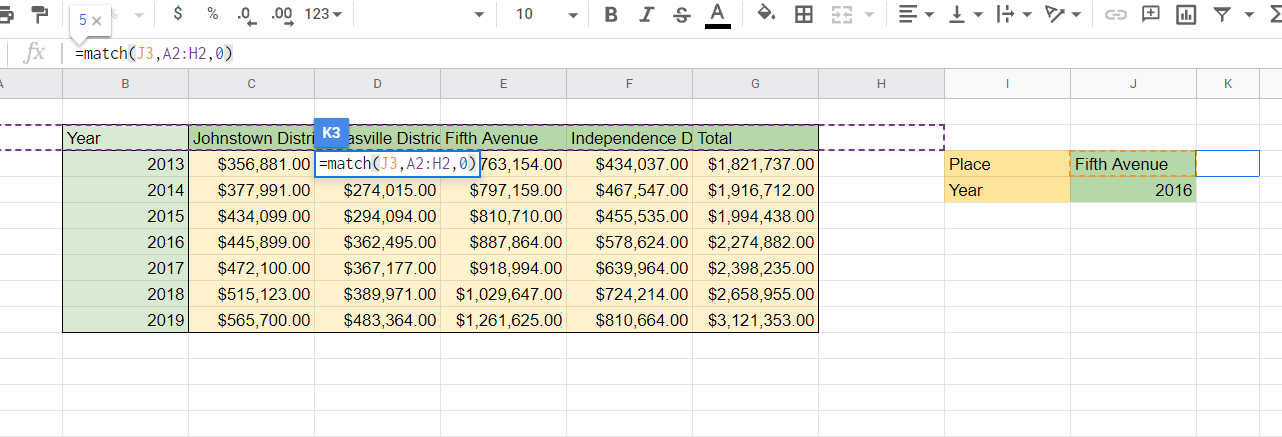How to use the MATCH function in Google Sheets

Learn how to use the MATCH function in Google Sheets. This is part 1 of a two-part series for designing a set of mini search bars in Google Sheets.1. How to use MATCH function
2. How to identify the column and row number of the given column and row header
3. Use the drop-down box alongside the MATCH function
4. Sample sheet8 Minutes

Let’s say we want to find the annual sales at a certain branch in a given year with the following data:

Specifically, we want to design a set of mini-search bars where we can type the row and column labels and then give us the value of a cell at their intersection. For example, we want to find the annual sales of the Fifth Avenue branch in 2016. The steps are as follows:

Step 1: Find the column number for the Fifth Avenue branch and the row number for the 2016 year.

Step 2: Convert the column and row numbers into a cell reference and then the value stored in it accessed.

This tutorial is the first part in the two-part tutorial in learning how to design a pair of search bars for this purpose. We will be doing Step 1 here using the MATCH function, and Step 2 uses the ADDRESS and INDIRECT functions. So, we will learn how to use the MATCH function, and then apply it to our data.

How to use MATCH function

The MATCH function is used to find the relative position of a string in a one-dimensional range. This means that the input array is either a single row or a single column. The syntax is as follows:

=MATCH(search_key, range, search_type)

Where search_key is the string to look for; range is the range of a single column or row to look for; and search_type tells Google Sheets how the entries are stored in the range. It can have three values:

1 : the entries are in ascending order

0 : the entries are not ordered; therefore an exact match must be done

-1 : the entries are in descending order.

We will use the following dummy ranges for our examples:

The search_key can be inserted inside quotation marks:

=match("Second",G5:G10,0)

Or stored in a cell, with the cell reference inserted in place of the original string:

=match(B8,G5:G10,0)

For our ultimate purpose, we can simply change the range to include the first cell in the column or row:

So the formula now looks like:

=match(B10,G1:G11,0)

Below are some of the forms of MATCH function used alongside their outputs:

You can tinker with the formulas by checking the sample sheet at the end of this tutorial.

How to identify the column and row number of the given column and row header

Let us now go back to our original target:

We now want to construct a pair of mini-search bars for the row and the column labels. First, allot areas for the search bars.

Here, we color-shaded the search bar labels with yellow-gold shade and the search bar themselves with light green shade. We have also added to the search bars the sample column label and row label.

Then, add the MATCH formulas to dedicated cells. For our example, we will add them to column K. Given the location of the column headers and row headers, we will first set the ranges to include the first cell of the column of the row headers.The range for the first MATCH function for the column label being set in the process.

The formula is:

=match(J3,A2:H2,0)

The same procedure will be done for the row headers:

The formula is:

=match(J4,B1:B9,0)

The final output is below, giving the row or column number in which the string is located:

For the next step, head over to our tutorial for the ADDRESS and INDIRECT functions to convert the column and row numbers to a cell reference and then display the content of that cell.

Use the drop-down box alongside the MATCH function

Instead of a search box, you can use a drop-down box as a source for the MATCH function. You can check this tutorial to learn how to add a drop-down box.

The advantage of a drop-down box is that it minimizes the spelling error when having long names as headers for either a column or a row.

Sample sheet

You can check the sample sheet below.

Oops! Something went wrong while submitting the form.

January 3, 2022

How to use the MATCH function in Google SheetsLet’s say we want to find the annual sales at a certain branch in a given year with the following data:

Specifically, we want to design a set of mini-search bars where we can type the row and column labels and then give us the value of a cell at their intersection. For example, we want to find the annual sales of the Fifth Avenue branch in 2016. The steps are as follows:

Step 1: Find the column number for the Fifth Avenue branch and the row number for the 2016 year.

Step 2: Convert the column and row numbers into a cell reference and then the value stored in it accessed.

This tutorial is the first part in the two-part tutorial in learning how to design a pair of search bars for this purpose. We will be doing Step 1 here using the MATCH function, and Step 2 uses the ADDRESS and INDIRECT functions. So, we will learn how to use the MATCH function, and then apply it to our data.

How to use MATCH function

The MATCH function is used to find the relative position of a string in a one-dimensional range. This means that the input array is either a single row or a single column. The syntax is as follows:

=MATCH(search_key, range, search_type)

Where search_key is the string to look for; range is the range of a single column or row to look for; and search_type tells Google Sheets how the entries are stored in the range. It can have three values:

1 : the entries are in ascending order

0 : the entries are not ordered; therefore an exact match must be done

-1 : the entries are in descending order.

We will use the following dummy ranges for our examples:

The search_key can be inserted inside quotation marks:

=match("Second",G5:G10,0)

Or stored in a cell, with the cell reference inserted in place of the original string:

=match(B8,G5:G10,0)

For our ultimate purpose, we can simply change the range to include the first cell in the column or row:

So the formula now looks like:

=match(B10,G1:G11,0)

Below are some of the forms of MATCH function used alongside their outputs:

You can tinker with the formulas by checking the sample sheet at the end of this tutorial.

How to identify the column and row number of the given column and row header

Let us now go back to our original target:

We now want to construct a pair of mini-search bars for the row and the column labels. First, allot areas for the search bars.

Here, we color-shaded the search bar labels with yellow-gold shade and the search bar themselves with light green shade. We have also added to the search bars the sample column label and row label.

Then, add the MATCH formulas to dedicated cells. For our example, we will add them to column K. Given the location of the column headers and row headers, we will first set the ranges to include the first cell of the column of the row headers.The range for the first MATCH function for the column label being set in the process.

The formula is:

=match(J3,A2:H2,0)

The same procedure will be done for the row headers:

The formula is:

=match(J4,B1:B9,0)

The final output is below, giving the row or column number in which the string is located:

For the next step, head over to our tutorial for the ADDRESS and INDIRECT functions to convert the column and row numbers to a cell reference and then display the content of that cell.

Use the drop-down box alongside the MATCH function

Instead of a search box, you can use a drop-down box as a source for the MATCH function. You can check this tutorial to learn how to add a drop-down box.

The advantage of a drop-down box is that it minimizes the spelling error when having long names as headers for either a column or a row.